# Factoring Polynomials

 Quizes today!

How do you compute something like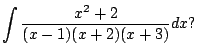So far you have no method for doing this. The trick (which is called partial fraction decomposition), is to write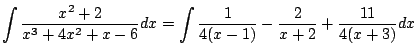(5.10)

The integral on the right is then easy to do (the answer involves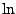's).

But how on earth do you right the rational function on the left hand side as a sum of the nice terms of the right hand side? Doing this is called partial fraction decomposition'', and it is a fundamental idea in mathematics. It relies on our ability to factor polynomials and saolve linear equations. As a first hint, notice that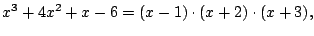so the denominators in the decomposition correspond to the factors of the denominator.

Before describing the secret behind (5.4.1), we'll discuss some background about how polynomials and rational functions work.

Theorem 5.4.1 (Fundamental Theorem of Algebra)   If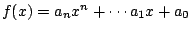is a polynomial, then there are complex numbers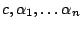such that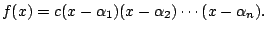Example 5.4.2   For example,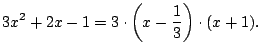And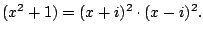If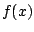is a polynomial, the roots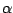of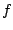correspond to the factors of. Thus if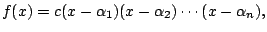then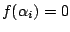for each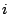(and nowhere else).

Definition 5.4.3 (Multiplicity of Zero)   The multiplicity of a zeroofis the number of times that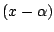appears as a factor of.

For example, if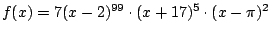, then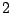is a zero with multiplicity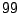,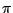is a zero with multiplicity, and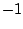is a zero multiplicity 0''.

Definition 5.4.4 (Rational Function)   A rational function is a quotient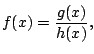where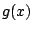and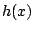are polynomials.

For example,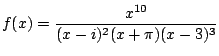(5.11)

is a rational function.

Definition 5.4.5 (Pole)   A pole of a rational functionis a complex numbersuch that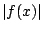is unbounded as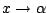.

For example, for (5.4.2) the poles are at,, and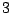. They have multiplicity,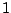, and, respectively.

William Stein 2006-03-15